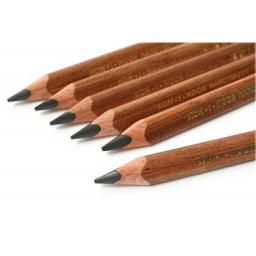# Sharpeners 4318

Pavel bought three pencils and a sharpener in a stationery store and paid 29 CZK. Jane ordered two pencils and two sharpeners, and her purchase price was CZK 46. How much cheaper is a pencil than a sharpener?

x =  17 Kc

### Step-by-step explanation:

3t+o=29
2t+2o=46
x = o-t

3·t+o=29
2·t+2·o=46
x = o-t

o+3t = 29
2o+2t = 46
o-t-x = 0

Pivot: Row 1 ↔ Row 2
2o+2t = 46
o+3t = 29
o-t-x = 0

Row 2 - 1/2 · Row 1 → Row 2
2o+2t = 46
2t = 6
o-t-x = 0

Row 3 - 1/2 · Row 1 → Row 3
2o+2t = 46
2t = 6
-2t-x = -23

Row 3 + Row 2 → Row 3
2o+2t = 46
2t = 6
-x = -17

x = -17/-1 = 17
t = 6/2 = 3
o = 46-2t/2 = 46-2 · 3/2 = 20

o = 20
t = 3
x = 17

Our linear equations calculator calculates it.Did you find an error or inaccuracy? Feel free to write us. Thank you!

Tips for related online calculators
Do you have a linear equation or system of equations and looking for its solution? Or do you have a quadratic equation?